﻿ Payment Calculations > Calculations Cost

# CALCULATIONS COST

Navegación:  Payment Calculations > Calculations Cost

# CALCULATIONS COST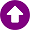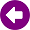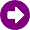Xirio  allows calculations of radio studios both free and pay. It is necessary that the user knows the limitations of free simulations, at low resolution, to decide what kind of calculation is best suited to each case. The following calculations are available available in Xirio, but not all are available as free calculation. LINK STUDY CALCULATIONS •Link Calculation: Available for free calculations at low resolutions. At high resolutions its cost is based on the pixels number to be calculated in addition to the cartography cost. •Digital Hop Report Calculation: Only available with payment method. When using UIT-R Rec.530 in calculation it is possible to obtain a digital hop report detailing quality and unavailability results of a link in fixed service. The cost of this calculation is fixed not depending on the link length or the cartography used. In free calculations a report with random data is obtained as example.COVERAGE STUDY CALCULATIONS •Coverage Calculation: Available for free calculations at low resolutions. At high resolutions its cost is based on the pixels number to be calculated in addition to the cost of cartography. •Coverage Statistics Calculation: Only available with payment calculation. To perform this calculation it is necessary to have previously calculated the coverage study at high resolution. Its cost is based on the coverage calculation cost since this cost is multiplied by a percentage that establishes the administrative layer used in the calculation to obtain the final calculation price.MULTICOVERAGE STUDY CALCULATIONS •Multi-coverage Calculation: Available for free calculations at low resolutions. At high resolutions its cost is based on the number of pixels to be calculated in addition to the cost of cartography used. In order to obtain the number of pixels to calculate, both pixels of coverage studies included in the multi-transmitter coverage and pixels of the whole area are computed: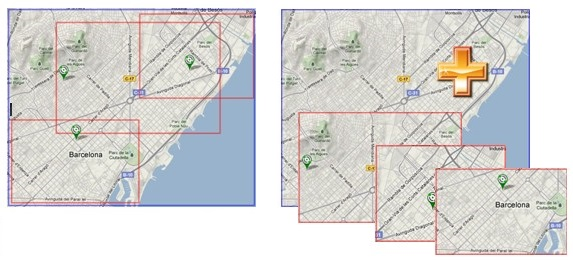•Multi-Coverage Statistics Calculation: Only available with payment calculation. To perform this calculation it is necessary to have previously calculated the coverage study at high resolution. Its cost is based on the coverage calculation cost since this cost is multiplied by a percentage that establishes the administrative layer used in the calculation to obtain the final calculation price.•Interference Calculation in the Multitransmitter study: Only available with payment calculation. Its cost is based on the pixels number to be calculated in addition to the cartography price. To obtain the number of pixels to calculate, both the coverage studies pixels included in the multi-coverage and the pixels of the whole area are computed.•Interference Statistics Calculation in the Multitransmitter study: Only available with payment calculation. In order to carry out this calculation, it is necessary to have previously calculated the interference in the multi-coverage study at high resolution. Its cost is based on the cost of calculating the interference in the multi-coverage study,  since this cost is multiplied by a percentage that establishes the administrative layer used in the calculation to obtain the final calculation price.•Punctual Calculation of interference in the Multitransmitter study: It is a free calculation but it is necessary to have previously calculated the interference study in the multi-coverage study.•Capacity Calculation only available in LTE services) in the Multitransmitter study:Only available with payment calculation. Its cost is based on the pixels number to be calculated in addition to the cartography price. In order to obtain the number of pixels to calculate, both pixels of coverage studies included in the multi-coverage and the pixels of the area as a whole are computed.•Route Calculation and Visualization in the Multitransmitter study: This calculation is free but it is necessary to have previously calculated the multi-coverage study or interference depending on the option that is selected:▪Generate the measurement display layer.▪Obtain signal value at the measurement points.▪Obtain interference value at the measurement points..  •Measurement Adjustment Calculation in the Multitransmitter study: This calculation is free but it is necessary to have previously performed a route calculation (option "Get signal value at the measurement points") of the multi-coverage study.TRANSPORT NETWORK STUDY CALCULATIONS •Transport Network Calculation: Only available with payment calculation, its cost is based on the pixels number to be calculated (the sum of all pixels that will be calculated for each link under study) in addition to the cartography price. If the "Consider interference" option is selected, the required pixels will be computed in the cost to calculate interference imposed at the link ends by the environment links.•Intervisibility Matrix Calculation: Only available with payment calculation, its cost is based on the pixels number needed to obtain the matrix.•Frequency Assignment Calculation: This calculation is free but it is necessary to have previously calculated interference in the multi-coverage study at high resolution by checking the option "Calculate compatibility matrices".EFFECTIVE HEIGHT STUDY CALCULATIONS •Height Effective Calculation: Only available for payment calculation, its cost is obtained from the total pixels on which is going to make the calculation, therefore this cost depends on the separation angle between each profile, in addition to the cartography cost.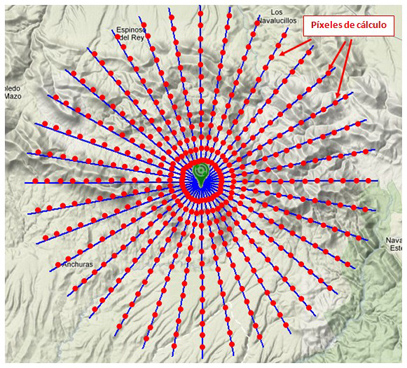When performing any payment calculation, the result will be stored for free for one month. It is also allowed to publish this result for a group of up to 10 users maximum. When making any free calculation, the result will be stored free of charge for three days. In this case it is not allowed to publish this result or export it in any way.